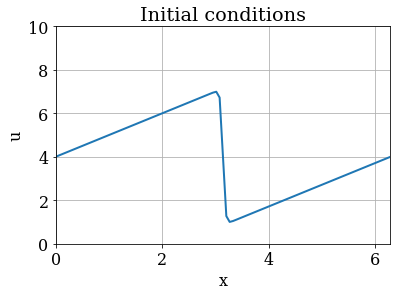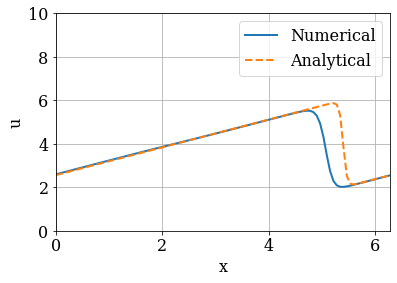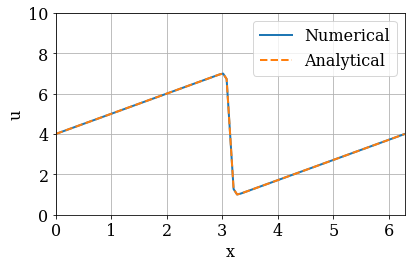# Space & Time¶

## Burgers' Equation¶

Hi there! We have reached the final lesson of the series Space and Time — Introduction to Finite-difference solutions of PDEs, the second module of "Practical Numerical Methods with Python".

We have learned about the finite-difference solution for the linear and non-linear convection equations and the diffusion equation. It's time to combine all these into one: Burgers' equation. The wonders of code reuse!

Before you continue, make sure you have completed the previous lessons of this series, it will make your life easier. You should have written your own versions of the codes in separate, clean Jupyter Notebooks or Python scripts.

$$\begin{equation} \frac{\partial u}{\partial t} + u \frac{\partial u}{\partial x} = \nu \frac{\partial ^2u}{\partial x^2} \end{equation}$$

As you can see, it is a combination of non-linear convection and diffusion. It is surprising how much you learn from this neat little equation!

We can discretize it using the methods we've already detailed in the previous notebooks of this module. Using forward difference for time, backward difference for space and our 2nd-order method for the second derivatives yields:

$$\begin{equation} \frac{u_i^{n+1}-u_i^n}{\Delta t} + u_i^n \frac{u_i^n - u_{i-1}^n}{\Delta x} = \nu \frac{u_{i+1}^n - 2u_i^n + u_{i-1}^n}{\Delta x^2} \end{equation}$$

As before, once we have an initial condition, the only unknown is $u_i^{n+1}$. We will step in time as follows:

$$\begin{equation} u_i^{n+1} = u_i^n - u_i^n \frac{\Delta t}{\Delta x} (u_i^n - u_{i-1}^n) + \nu \frac{\Delta t}{\Delta x^2}(u_{i+1}^n - 2u_i^n + u_{i-1}^n) \end{equation}$$

### Initial and Boundary Conditions¶

To examine some interesting properties of Burgers' equation, it is helpful to use different initial and boundary conditions than we've been using for previous steps.

The initial condition for this problem is going to be:

$$\begin{eqnarray} u &=& -\frac{2 \nu}{\phi} \frac{\partial \phi}{\partial x} + 4 \\\ \phi(t=0) = \phi_0 &=& \exp \bigg(\frac{-x^2}{4 \nu} \bigg) + \exp \bigg(\frac{-(x-2 \pi)^2}{4 \nu} \bigg) \end{eqnarray}$$

This has an analytical solution, given by:

$$\begin{eqnarray} u &=& -\frac{2 \nu}{\phi} \frac{\partial \phi}{\partial x} + 4 \\\ \phi &=& \exp \bigg(\frac{-(x-4t)^2}{4 \nu (t+1)} \bigg) + \exp \bigg(\frac{-(x-4t -2 \pi)^2}{4 \nu(t+1)} \bigg) \end{eqnarray}$$

The boundary condition will be:

$$\begin{equation} u(0) = u(2\pi) \end{equation}$$

This is called a periodic boundary condition. Pay attention! This will cause you a bit of headache if you don't tread carefully.

### Saving Time with SymPy¶

The initial condition we're using for Burgers' Equation can be a bit of a pain to evaluate by hand. The derivative $\frac{\partial \phi}{\partial x}$ isn't too terribly difficult, but it would be easy to drop a sign or forget a factor of $x$ somewhere, so we're going to use SymPy to help us out.

SymPy is the symbolic math library for Python. It has a lot of the same symbolic math functionality as Mathematica with the added benefit that we can easily translate its results back into our Python calculations (it is also free and open source).

Start by loading the SymPy library, together with our favorite library, NumPy.

In :
import numpy
import sympy
from matplotlib import pyplot
%matplotlib inline

In :
# Set the font family and size to use for Matplotlib figures.
pyplot.rcParams['font.family'] = 'serif'
pyplot.rcParams['font.size'] = 16


We're also going to tell SymPy that we want all of its output to be rendered using $\LaTeX$. This will make our Notebook beautiful!

In :
sympy.init_printing()


Start by setting up symbolic variables for the three variables in our initial condition. It's important to recognize that once we've defined these symbolic variables, they function differently than "regular" Python variables.

If we type x into a code block, we'll get an error:

In :
x

---------------------------------------------------------------------------
NameError                                 Traceback (most recent call last)
<ipython-input-4-6fcf9dfbd479> in <module>
----> 1 x

NameError: name 'x' is not defined

x is not defined, so this shouldn't be a surprise. Now, let's set up x as a symbolic variable:

In :
x = sympy.symbols('x')


Now let's see what happens when we type x into a code cell:

In :
x

Out:
$\displaystyle x$

The value of x is $x$. Sympy is also referred to as a computer algebra system -- normally the value of 5*x will return the product of 5 and whatever value x is pointing to. But, if we define x as a symbol, then something else happens:

In :
5 * x

Out:
$\displaystyle 5 x$

This will let us manipulate an equation with unknowns using Python! Let's start by defining symbols for $x$, $\nu$ and $t$ and then type out the full equation for $\phi$. We should get a nicely rendered version of our $\phi$ equation.

In :
x, nu, t = sympy.symbols('x nu t')
phi = (sympy.exp(-(x - 4 * t)**2 / (4 * nu * (t + 1))) +
sympy.exp(-(x - 4 * t - 2 * sympy.pi)**2 / (4 * nu * (t + 1))))
phi

Out:
$\displaystyle e^{- \frac{\left(- 4 t + x - 2 \pi\right)^{2}}{4 \nu \left(t + 1\right)}} + e^{- \frac{\left(- 4 t + x\right)^{2}}{4 \nu \left(t + 1\right)}}$

It's maybe a little small, but that looks right. Now to evaluate our partial derivative $\frac{\partial \phi}{\partial x}$ is a trivial task. To take a derivative with respect to $x$, we can just use:

In :
phiprime = phi.diff(x)
phiprime

Out:
$\displaystyle - \frac{\left(- 8 t + 2 x\right) e^{- \frac{\left(- 4 t + x\right)^{2}}{4 \nu \left(t + 1\right)}}}{4 \nu \left(t + 1\right)} - \frac{\left(- 8 t + 2 x - 4 \pi\right) e^{- \frac{\left(- 4 t + x - 2 \pi\right)^{2}}{4 \nu \left(t + 1\right)}}}{4 \nu \left(t + 1\right)}$

If you want to see the non-rendered version, just use the Python print command.

In :
print(phiprime)

-(-8*t + 2*x)*exp(-(-4*t + x)**2/(4*nu*(t + 1)))/(4*nu*(t + 1)) - (-8*t + 2*x - 4*pi)*exp(-(-4*t + x - 2*pi)**2/(4*nu*(t + 1)))/(4*nu*(t + 1))


### Now what?¶

Now that we have the Pythonic version of our derivative, we can finish writing out the full initial condition equation and then translate it into a usable Python expression. For this, we'll use the lambdify function, which takes a SymPy symbolic equation and turns it into a callable function.

In :
from sympy.utilities.lambdify import lambdify

u = -2 * nu * (phiprime / phi) + 4
print(u)

-2*nu*(-(-8*t + 2*x)*exp(-(-4*t + x)**2/(4*nu*(t + 1)))/(4*nu*(t + 1)) - (-8*t + 2*x - 4*pi)*exp(-(-4*t + x - 2*pi)**2/(4*nu*(t + 1)))/(4*nu*(t + 1)))/(exp(-(-4*t + x - 2*pi)**2/(4*nu*(t + 1))) + exp(-(-4*t + x)**2/(4*nu*(t + 1)))) + 4


### Lambdify¶

To lambdify this expression into a usable function, we tell lambdify which variables to request and the function we want to plug them into.

In :
u_lamb = lambdify((t, x, nu), u)
print('The value of u at t=1, x=4, nu=3 is {}'.format(u_lamb(1, 4, 3)))

The value of u at t=1, x=4, nu=3 is 3.49170664206445


### Back to Burgers' Equation¶

Now that we have the initial conditions set up, we can proceed and finish setting up the problem. We can generate the plot of the initial condition using our lambdify-ed function.

In :
# Set parameters.
nx = 101  # number of spatial grid points
L = 2.0 * numpy.pi  # length of the domain
dx = L / (nx - 1)  # spatial grid size
nu = 0.07  # viscosity
nt = 100  # number of time steps to compute
sigma = 0.1  # CFL limit
dt = sigma * dx**2 / nu  # time-step size

# Discretize the domain.
x = numpy.linspace(0.0, L, num=nx)


We have a function u_lamb but we need to create an array u0 with our initial conditions. u_lamb will return the value for any given time $t$, position $x$ and $nu$. We can use a for-loop to cycle through values of x to generate the u0 array. That code would look something like this:

u0 = numpy.empty(nx)

for i, x0 in enumerate(x):
u0[i] = u_lamb(t, x0, nu)


But there's a cleaner, more beautiful way to do this -- list comprehension.

We can create a list of all of the appropriate u values by typing

[u_lamb(t, x0, nu) for x0 in x]


You can see that the syntax is similar to the for-loop, but it only takes one line. Using a list comprehension will create... a list. This is different from an array, but converting a list to an array is trivial using numpy.asarray().

With the list comprehension in place, the three lines of code above become one:

u = numpy.asarray([u_lamb(t, x0, nu) for x0 in x])

In :
# Set initial conditions.
t = 0.0
u0 = numpy.array([u_lamb(t, xi, nu) for xi in x])
u0

Out:
array([4.        , 4.06283185, 4.12566371, 4.18849556, 4.25132741,
4.31415927, 4.37699112, 4.43982297, 4.50265482, 4.56548668,
4.62831853, 4.69115038, 4.75398224, 4.81681409, 4.87964594,
4.9424778 , 5.00530965, 5.0681415 , 5.13097336, 5.19380521,
5.25663706, 5.31946891, 5.38230077, 5.44513262, 5.50796447,
5.57079633, 5.63362818, 5.69646003, 5.75929189, 5.82212374,
5.88495559, 5.94778745, 6.0106193 , 6.07345115, 6.136283  ,
6.19911486, 6.26194671, 6.32477856, 6.38761042, 6.45044227,
6.51327412, 6.57610598, 6.63893783, 6.70176967, 6.76460125,
6.82742866, 6.89018589, 6.95176632, 6.99367964, 6.72527549,
4.        , 1.27472451, 1.00632036, 1.04823368, 1.10981411,
1.17257134, 1.23539875, 1.29823033, 1.36106217, 1.42389402,
1.48672588, 1.54955773, 1.61238958, 1.67522144, 1.73805329,
1.80088514, 1.863717  , 1.92654885, 1.9893807 , 2.05221255,
2.11504441, 2.17787626, 2.24070811, 2.30353997, 2.36637182,
2.42920367, 2.49203553, 2.55486738, 2.61769923, 2.68053109,
2.74336294, 2.80619479, 2.86902664, 2.9318585 , 2.99469035,
3.0575222 , 3.12035406, 3.18318591, 3.24601776, 3.30884962,
3.37168147, 3.43451332, 3.49734518, 3.56017703, 3.62300888,
3.68584073, 3.74867259, 3.81150444, 3.87433629, 3.93716815,
4.        ])

Now that we have the initial conditions set up, we can plot it to see what $u(x,0)$ looks like:

In :
# Plot the initial conditions.
pyplot.figure(figsize=(6.0, 4.0))
pyplot.title('Initial conditions')
pyplot.xlabel('x')
pyplot.ylabel('u')
pyplot.grid()
pyplot.plot(x, u0, color='C0', linestyle='-', linewidth=2)
pyplot.xlim(0.0, L)
pyplot.ylim(0.0, 10.0);This is definitely not the hat function we've been dealing with until now. We call it a "saw-tooth function". Let's proceed forward and see what happens.

### Periodic Boundary Conditions¶

We will implement Burgers' equation with periodic boundary conditions. If you experiment with the linear and non-linear convection notebooks and make the simulation run longer (by increasing nt) you will notice that the wave will keep moving to the right until it no longer even shows up in the plot.

With periodic boundary conditions, when a point gets to the right-hand side of the frame, it wraps around back to the front of the frame.

Recall the discretization that we worked out at the beginning of this notebook:

$$\begin{equation} u_i^{n+1} = u_i^n - u_i^n \frac{\Delta t}{\Delta x} (u_i^n - u_{i-1}^n) + \nu \frac{\Delta t}{\Delta x^2}(u_{i+1}^n - 2u_i^n + u_{i-1}^n) \end{equation}$$

What does $u_{i+1}^n$ mean when $i$ is already at the end of the frame?

In :
# Integrate the Burgers' equation in time.
u = u0.copy()
for n in range(nt):
un = u.copy()
# Update all interior points.
u[1:-1] = (un[1:-1] -
un[1:-1] * dt / dx * (un[1:-1] - un[:-2]) +
nu * dt / dx**2 * (un[2:] - 2 * un[1:-1] + un[:-2]))
# Update boundary points.
u = (un -
un * dt / dx * (un - un[-1]) +
nu * dt / dx**2 * (un - 2 * un + un[-1]))
u[-1] = (un[-1] -
un[-1] * dt / dx * (un[-1] - un[-2]) +
nu * dt / dx**2 * (un - 2 * un[-1] + un[-2]))

In :
# Compute the analytical solution.
u_analytical = numpy.array([u_lamb(nt * dt, xi, nu) for xi in x])

In :
# Plot the numerical solution along with the analytical solution.
pyplot.figure(figsize=(6.0, 4.0))
pyplot.xlabel('x')
pyplot.ylabel('u')
pyplot.grid()
pyplot.plot(x, u, label='Numerical',
color='C0', linestyle='-', linewidth=2)
pyplot.plot(x, u_analytical, label='Analytical',
color='C1', linestyle='--', linewidth=2)
pyplot.legend()
pyplot.xlim(0.0, L)
pyplot.ylim(0.0, 10.0);Let's now create an animation with the animation module of Matplotlib to observe how the numerical solution changes over time compared to the analytical solution. We start by importing the module from Matplotlib as well as the special HTML display method.

In :
from matplotlib import animation
from IPython.display import HTML


We create a function burgers to computes the numerical solution of the 1D Burgers' equation over time. (The function returns the history of the solution: a list with nt elements, each one being the solution in the domain at a time step.)

In :
def burgers(u0, dx, dt, nu, nt=20):
"""
Computes the numerical solution of the 1D Burgers' equation
over the time steps.

Parameters
----------
u0 : numpy.ndarray
The initial conditions as a 1D array of floats.
dx : float
The grid spacing.
dt : float
The time-step size.
nu : float
The viscosity.
nt : integer, optional
The number of time steps to compute;
default: 20.

Returns
-------
u_hist : list of numpy.ndarray objects
The history of the numerical solution.
"""
u_hist = [u0.copy()]
u = u0.copy()
for n in range(nt):
un = u.copy()
# Update all interior points.
u[1:-1] = (un[1:-1] -
un[1:-1] * dt / dx * (un[1:-1] - un[:-2]) +
nu * dt / dx**2 * (un[2:] - 2 * un[1:-1] + un[:-2]))
# Update boundary points.
u = (un -
un * dt / dx * (un - un[-1]) +
nu * dt / dx**2 * (un - 2 * un + un[-1]))
u[-1] = (un[-1] -
un[-1] * dt / dx * (un[-1] - un[-2]) +
nu * dt / dx**2 * (un - 2 * un[-1] + un[-2]))
u_hist.append(u.copy())
return u_hist

In :
# Compute the history of the numerical solution.
u_hist = burgers(u0, dx, dt, nu, nt=nt)

In :
# Compute the history of the analytical solution.
u_analytical = [numpy.array([u_lamb(n * dt, xi, nu) for xi in x])
for n in range(nt)]

In :
fig = pyplot.figure(figsize=(6.0, 4.0))
pyplot.xlabel('x')
pyplot.ylabel('u')
pyplot.grid()
u0_analytical = numpy.array([u_lamb(0.0, xi, nu) for xi in x])
line1 = pyplot.plot(x, u0, label='Numerical',
color='C0', linestyle='-', linewidth=2)
line2 = pyplot.plot(x, u0_analytical, label='Analytical',
color='C1', linestyle='--', linewidth=2)
pyplot.legend()
pyplot.xlim(0.0, L)
pyplot.ylim(0.0, 10.0)
fig.tight_layout()In :
def update_plot(n, u_hist, u_analytical):
"""
Update the lines y-data of the Matplotlib figure.

Parameters
----------
n : integer
The time-step index.
u_hist : list of numpy.ndarray objects
The history of the numerical solution.
u_analytical : list of numpy.ndarray objects
The history of the analytical solution.
"""
fig.suptitle('Time step {:0>2}'.format(n))
line1.set_ydata(u_hist[n])
line2.set_ydata(u_analytical[n])

In :
# Create an animation.
anim = animation.FuncAnimation(fig, update_plot,
frames=nt, fargs=(u_hist, u_analytical),
interval=100)

In :
# Display the video.
HTML(anim.to_html5_video())

Out:

## Array Operation Speed Increase¶

Coding up discretization schemes using array operations can be a bit of a pain. It requires much more mental effort on the front-end than using two nested for loops. So why do we do it? Because it's fast. Very, very fast.

Here's what the Burgers code looks like using two nested for loops. It's easier to write out, plus we only have to add one "special" condition to implement the periodic boundaries.

At the top of the cell, you'll see the decorator %%timeit. This is called a "cell magic". It runs the cell several times and returns the average execution time for the contained code.

Let's see how long the nested for loops take to finish.

In :
%%timeit
# Set initial conditions.
u = numpy.array([u_lamb(t, x0, nu) for x0 in x])
# Integrate in time using a nested for loop.
for n in range(nt):
un = u.copy()
# Update all interior points and the left boundary point.
for i in range(nx - 1):
u[i] = (un[i] -
un[i] * dt / dx *(un[i] - un[i - 1]) +
nu * dt / dx**2 * (un[i + 1] - 2 * un[i] + un[i - 1]))
# Update the right boundary.
u[-1] = (un[-1] -
un[-1] * dt / dx * (un[-1] - un[-2]) +
nu * dt / dx**2 * (un- 2 * un[-1] + un[-2]))

23.1 ms ± 344 µs per loop (mean ± std. dev. of 7 runs, 10 loops each)


Less than 50 milliseconds. Not bad, really.

Now let's look at the array operations code cell. Notice that we haven't changed anything, except we've added the %%timeit magic and we're also resetting the array u to its initial conditions.

This takes longer to code and we have to add two special conditions to take care of the periodic boundaries. Was it worth it?

In :
%%timeit
# Set initial conditions.
u = numpy.array([u_lamb(t, xi, nu) for xi in x])
# Integrate in time using array operations.
for n in range(nt):
un = u.copy()
# Update all interior points.
u[1:-1] = (un[1:-1] -
un[1:-1] * dt / dx * (un[1:-1] - un[:-2]) +
nu * dt / dx**2 * (un[2:] - 2 * un[1:-1] + un[:-2]))
# Update boundary points.
u = (un -
un * dt / dx * (un - un[-1]) +
nu * dt / dx**2 * (un - 2 * un + un[-1]))
u[-1] = (un[-1] -
un[-1] * dt / dx * (un[-1] - un[-2]) +
nu * dt / dx**2 * (un - 2 * un[-1] + un[-2]))

2.52 ms ± 64.7 µs per loop (mean ± std. dev. of 7 runs, 100 loops each)


Yes, it is absolutely worth it. That's a nine-fold speed increase. For this exercise, you probably won't miss the extra 40 milliseconds if you use the nested for loops, but what about a simulation that has to run through millions and millions of iterations? Then that little extra effort at the beginning will definitely pay off.

###### The cell below loads the style of the notebook.¶
In :
from IPython.core.display import HTML
css_file = '../../styles/numericalmoocstyle.css'